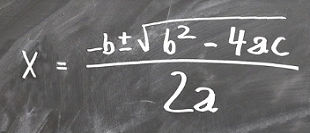Search IntMath
Close

# Basic Algebra

### Also on IntMath

Related algebra chapters:This chapter contains elementary algebra tutorials on the following topics:

1. Adding and Subtracting Algebraic Expressions, shows you how to do problems like: Simplify: −2[−3(x − 2y) + 4y].

2. Multiplication of algebra expressions, has examples like:
Expand (2x + 3)(x2x − 5).

3. Division of algebraic expressions, for example: (12a2b) ÷ (3ab2)

4. Solving Equations, like this one: 5 − (x + 2) = 5x.

5. Formulas and Literal Equations, which shows how to solve an equation for a particular variable.

6. Applied Verbal Problems shows why we are doing all this.

## What is Algebra?

Algebra is the branch of mathematics that uses letters in place of some unknown numbers.

You've been using algebra since your early schooling, when you learned formulas like the area of a rectangle, with width w, height h:

A = w × h

We used letters to stand for numbers. Once we knew the width and height, we could substitute them into the formula and find our area.

Another one you may have seen is the area of a circle, with radius r:

A = πr2

As soon as we know the length of the sides, we can find the area.

Literal numbers (the letters used in algebra) can either stand for variables (the value of the letter can change, like the w, h and r in the examples of the area of a rectangle and the area of a circle) or constants (where the value does not change), for example:

π (the ratio circumference/diameter of a circle, value 3.141592....)

g (the accelaration due to gravity, 9.8 m/s2),

e (which has a constant value of 2.781828...).

And as my students constantly ask...

## Why Do We Have to do This?

Algebra is a powerful tool for problem solving in science, engineering, economics, finance, architecture, ship-building and many other day-to-day tasks.

If we didn't use letters in place of numbers (and used words instead), we would be writing many pages for each problem and it would be much more confusing.

This elementary algebra chapter follows on from the earlier chapter on Numbers.

### If you find this chapter difficult...

If you struggle with this chapter, it may be a good idea to go back and remind yourself about basic number properties first, since that's important background.

### On with the show

OK, let's move on and learn some basic algebra tips:

## Problem SolverThis tool combines the power of mathematical computation engine that excels at solving mathematical formulas with the power of GPT large language models to parse and generate natural language. This creates math problem solver thats more accurate than ChatGPT, more flexible than a calculator, and faster answers than a human tutor. Learn More.